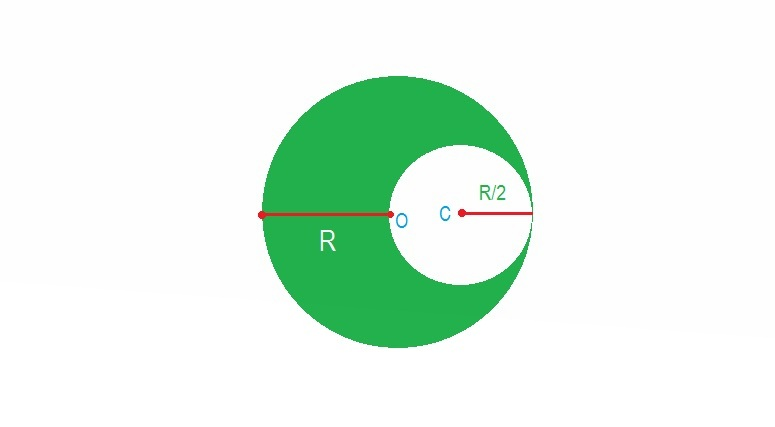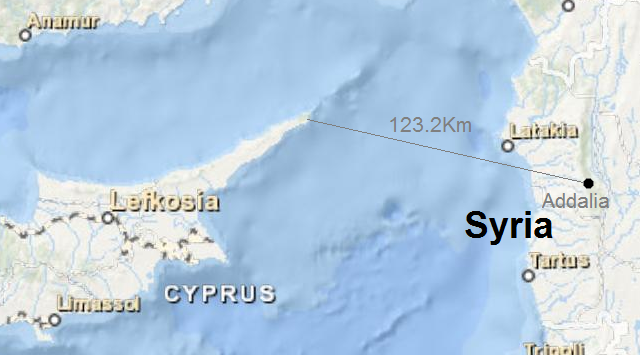Classical Mechanics

# Mechanics Warmups: Level 3 ChallengesA frog sits on the end of a long board of length $L=5$ m. The board rests on a frictionless horizontal table. The frog wants to jump to the opposite end of the board.

What is the minimum take-off speed (in m/s) relative to ground that allows the frog to complete the trick?

Assumptions and Details

• The board and the frog have equal masses.
• The frog's feet do not slip on the board.
• Let $g=\SI[per-mode=symbol]{9.8}{\meter\per\second\squared}$.My Fermi estimate for the amount of data comprising Brilliant.org is 215 GB. Suppose that all of this data is stored on SSD flash drives, which currently require about $10^4$ electrons per 1 bit, and that this data is information rich (Hint: a hard drive of all 1s or all 0s contains zero information). Under these assumptions, what is the mass (in kilograms) of the data that makes up Brilliant.org?

Details

• $\text{Vol(DB)} = 15 \text{ GB content} + 200 \text{ GB activity} = 215 \text{ GB data}$
• $8\text{ bits} = 1\text{ byte}$
• $1,073,741,824\text{ bytes} = 1\text{ gigabyte}$A solid sphere of uniform density with mass $M$ and radius $R$ has its center at $(0,0,0)$ which is the origin of the coordinate system. A spherical cavity of radius $\frac { R }{ 2 }$ having center at $C$ is made in the sphere by removing part of the material as shown in the figure above. Then the gravitational field intensity at $C$ is

Four beetles, call them John, Paul, George, and Ringo, start off at the corners of a square room 2 meters on a side. Looking down into the room from above, John is in the upper left, Paul in the upper right, George in the lower right, and Ringo in the lower left. The beetles start crawling towards the next beetle, i.e. John crawls directly at Paul, Paul crawls directly at George, George crawls directly at Ringo, and Ringo crawls directly at John. Each beetle's speed is $0.01~\mbox{m/s}$.

How far has one beetle traveled in meters when all the beetles meet in the middle?

Addalia is a big town near Jableh at western Syria, It's located near a mountain, and people who live in Addalia say that on a sunny clear day they can see the island of Cyprus from the top of the mountain. In this problem, we're going to determine if they can really see Cyprus, and how high they need to be on the mountain to begin to see the coast of Cyprus.Find $h$, the viewing height (in m) required to see Cyprus from Addalia.

Details and assumptions:

• Assume that the earth is completely spherical.
• The straight line distance between Addalia and the Cyprus coastline 123.2km.
• The Earth radius is 6400 km.
• Addalia's mountain is 1083.5m high.
• Note the height could be more than the height of the mountain, i.e. if $h\leq1083.5$ m, the coast can't be seen.
• Assume light travels in a straight line.
×